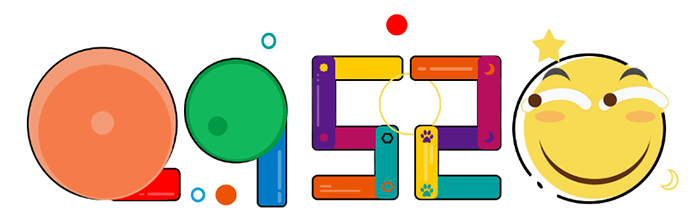# 几道PHP试题，来试试看看你会不会也掉入陷阱### 第一题

``````\$arr = array(0=>1,"aa"=>2, 3, 4);
foreach(\$arr as \$key=>\$val){
print(\$key == "aa" ? 5 : \$val);
}``````

``````Array
(
 => 1
[aa] => 2
 => 3
 => 4
)``````

``print(\$key === "aa" ? 5 : \$val);``

### 第二题

``````\$i='11';
printf("%d\n",printf("%d",printf("%d",\$i)));``````

``var_dump(printf("%d",\$i));``

### 第三题

``````\$a = 3;
\$b = 5;
if(\$a = 5 || \$b = 7) {
\$a++;
\$b++;
}
echo \$a . " " . \$b;``````

### 第四题

``````\$count = 5;
function get_count() {
static \$count = 0;
return \$count++;
}
++\$count;
get_count();
echo get_count();``````

### 第五题

``````\$a = count ("567")  + count(null) + count(false);
echo \$a;``````

## 2 条评论

•PHP小白

•@里维斯社 哈哈